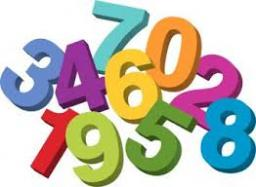# Reduced 1631

Three times the number reduced by 10 is as much as 100, as 100 is more than twice that. What is the number?

x =  42

### Step-by-step explanation:

(3x-10) -100 = 100 - 2x

(3·x-10) -100 = 100 - 2·x

5x = 210

x = 210/5 = 42

x = 42

Our simple equation calculator calculates it.Did you find an error or inaccuracy? Feel free to write us. Thank you!

Tips for related online calculators
Do you have a linear equation or system of equations and looking for its solution? Or do you have a quadratic equation?